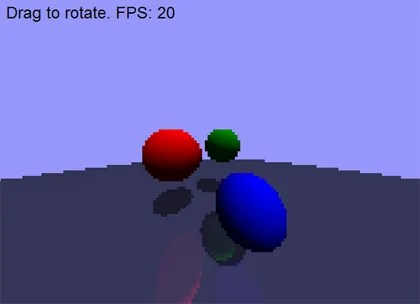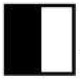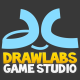## AS3 Real-Time Raytracing

Forrest Briggs throwing down with a real-time raytracer in AS3. Also a C++ OpenGL version sample on the page.

Real-time pixel manipulation in flash is getting faster, but is still probably going to have to be faked in AS3, maybe AS4 will provide us per pixel speeds that Andre Michelle has been harping on since flash 8.5. Native operations can be much faster in that area. AIF might look to change some of that but that is Flash 10.Here is the code for the as3 raytracer. Read more at laserpirate.

package
{
import flash.display.Sprite;
import flash.display.Bitmap;
import flash.display.BitmapData;
import flash.events.Event;
import flash.utils.getTimer;
import flash.events.MouseEvent;
import flash.text.TextField;
import flash.text.TextFormat;

public class RayTracer extends Sprite
{
private var t:Number;
private var dt:Number = .01;
private var frameTimeTxt:TextField;

public static const BUFFER_WIDTH:int = 160;
public static const BUFFER_HEIGHT:int = 120;
public static const BUFFER_SCALEDDOWN:int = 320 / BUFFER_WIDTH;

public static const HALF_BUFFER_WIDTH:int = BUFFER_WIDTH / 2;
public static const HALF_BUFFER_HEIGHT:int = BUFFER_HEIGHT / 2;

private var outputBitmapData:BitmapData;
private var outputBitmap:Bitmap;

public var FOV:Number = 20;

public var sphereCenterX:Array = [0, 0, 0, 0];
public var sphereCenterY:Array = [0, -.2, .4, 100.5];
public var sphereCenterZ:Array = [4, 4, 4, 10];
public var sphereRadius:Array = [.35, .35, .25, 100];
public var sphereR:Array = [255, 0, 0, 20];
public var sphereG:Array = [0, 150, 0, 20];
public var sphereB:Array = [0, 0, 255, 20];
public var sphereReflects:Array = [false, false, false, true];
public var sphereReflectiveness:Array = [0,0,0,.3];
public var sphere2dX:Array = new Array(sphereCenterX.length);
public var sphere2dY:Array = new Array(sphereCenterX.length);
public var sphere2dR:Array = new Array(sphereCenterX.length);

public var numSpheres = sphereCenterX.length;

var skyR:int = 20;
var skyG:int = 20;
var skyB:int = 20;
var skyColor:int = (skyR< <16) + (skyG<<8) + skyB; var ambientIllumination:Number = .1; var canvas:BlankClip; var theta:Number = 0; var mouseIsDown:Boolean = false; var mouseDownTheta:Number = 0; var mouseDownX:Number = 0; public function RayTracer() { outputBitmapData = new BitmapData(BUFFER_WIDTH, BUFFER_HEIGHT, false); outputBitmap = new Bitmap(outputBitmapData); addChild(outputBitmap); //outputBitmap.smoothing = true; outputBitmap.width= 320; outputBitmap.height = 240; canvas = new BlankClip; addChild(canvas); canvas.buttonMode = true; canvas.useHandCursor = true; frameTimeTxt = new TextField(); frameTimeTxt.defaultTextFormat = new TextFormat("Arial"); frameTimeTxt.x = 8; frameTimeTxt.y = 8; frameTimeTxt.width = 640; frameTimeTxt.textColor = 0xFFFFFF; frameTimeTxt.selectable = false; addChild(frameTimeTxt); t = 0; addEventListener(Event.ENTER_FRAME, update, false, 0, true); canvas.addEventListener(MouseEvent.MOUSE_DOWN, mouseDownHandler); canvas.addEventListener(MouseEvent.MOUSE_UP, mouseUpHandler); } public function mouseDownHandler(e:*):void { mouseIsDown = true; mouseDownX = stage.mouseX; mouseDownTheta = theta; } public function mouseUpHandler(e:*):void { mouseIsDown = false; } public function update(e:*) { // start frame timer and update global time var timer:Number = getTimer(); t += dt; // handle mouse rotation if( mouseIsDown ) theta = mouseDownTheta - .0015 * (stage.mouseX - mouseDownX); theta += dt; // do some funky animation sphereCenterX = .5*Math.sin(theta*5); sphereCenterZ =1 + .5*Math.cos(theta*5); sphereCenterX = .5*Math.sin(theta*5 + 2 * Math.PI / 3); sphereCenterZ = 1 + .5*Math.cos(theta*5 + 2 * Math.PI / 3); sphereCenterX = .5*Math.sin(theta*5 + 4 * Math.PI / 3); sphereCenterZ = 1 + .5*Math.cos(theta*5 + 4 * Math.PI / 3); // reused variables var x:int; var y:int; var i:int; var j:int; var r:int; var g:int; var b:int; var dx:Number; var dy:Number; var rayDirX:Number; var rayDirY:Number; var rayDirZ:Number; var rayDirMag:Number; var reflectRayDirX:Number; var reflectRayDirY:Number; var reflectRayDirZ:Number; var intersectionX:Number; var intersectionY:Number; var intersectionZ:Number; var reflectIntersectionX:Number; var reflectIntersectionY:Number; var reflectIntersectionZ:Number; var rayToSphereCenterX:Number; var rayToSphereCenterY:Number; var rayToSphereCenterZ:Number; var lengthRTSC2:Number; var closestApproach:Number; var halfCord2:Number; var dist:Number; var normalX:Number; var normalY:Number; var normalZ:Number; var normalMag:Number; var illumination:Number; var reflectIllumination:Number; var reflectR:Number; var reflectG:Number; var reflectB:Number; // setup light dir var lightDirX:Number = .3; var lightDirY:Number = -1; var lightDirZ:Number = -.5; var lightDirMag:Number = 1/Math.sqrt(lightDirX*lightDirX +lightDirY*lightDirY +lightDirZ*lightDirZ); lightDirX *= lightDirMag; lightDirY *= lightDirMag; lightDirZ *= lightDirMag; // vars used to in intersection tests var closestIntersectionDist:Number; var closestSphereIndex:int; var reflectClosestSphereIndex:int; // compute screen space bounding circles //canvas.graphics.clear(); //canvas.graphics.lineStyle(1, 0xFF0000, .25); for(i = 0; i < numSpheres; ++i) { sphere2dX[i] = (BUFFER_WIDTH / 2 + FOV * sphereCenterX[i] / sphereCenterZ[i]); sphere2dY[i] = (BUFFER_HEIGHT /2 + FOV * sphereCenterY[i] / sphereCenterZ[i]); sphere2dR[i] = (3 * FOV * sphereRadius[i] / sphereCenterZ[i]); //canvas.graphics.drawCircle(sphere2dX[i]*BUFFER_SCALEDDOWN, sphere2dY[i]*BUFFER_SCALEDDOWN, sphere2dR[i]*BUFFER_SCALEDDOWN); sphere2dR[i] *= sphere2dR[i]; // store the squared value } // write to each pixel outputBitmapData.lock(); for(y = 0; y < BUFFER_HEIGHT; ++y) { for(x = 0; x < BUFFER_WIDTH; ++x) { // compute ray direction rayDirX = x - HALF_BUFFER_WIDTH; rayDirY = y - HALF_BUFFER_HEIGHT; rayDirZ = FOV; rayDirMag = 1/Math.sqrt(rayDirX * rayDirX + rayDirY * rayDirY +rayDirZ * rayDirZ); rayDirX *= rayDirMag; rayDirY *= rayDirMag; rayDirZ *= rayDirMag; /// trace the primary ray /// closestIntersectionDist = Number.POSITIVE_INFINITY; closestSphereIndex = -1 for(i = 0; i < numSpheres; ++i) { // check against screen space bounding circle dx = x - sphere2dX[i]; dy = y - sphere2dY[i]; if( dx * dx + dy * dy > sphere2dR[i] ) continue;

// begin actual ray tracing if its inside the bounding circle

lengthRTSC2 = sphereCenterX[i] * sphereCenterX[i] +
sphereCenterY[i] * sphereCenterY[i] +
sphereCenterZ[i] * sphereCenterZ[i];

closestApproach = sphereCenterX[i] * rayDirX +
sphereCenterY[i] * rayDirY +
sphereCenterZ[i] * rayDirZ;

if( g > 255 ) g = 255;
if( b > 255 ) b = 255;

} // end if reflected ray hits

} /// end if reflects
else // primary ray doesn’t reflect
{
r = sphereR[closestSphereIndex] * illumination;
g = sphereG[closestSphereIndex] * illumination;
b = sphereB[closestSphereIndex] * illumination;
}

outputBitmapData.setPixel(x, y, (r<<16) + (g<<8) + b); } // end if primary ray hit } // end x loop } // end y loop outputBitmapData.unlock(); // compute FPS var fps:Number = 1.0/((getTimer() - timer) / 1000.0); frameTimeTxt.text = "Drag to rotate. FPS: " + int(fps); } } }[/sourcecode]

### 3 Responses to “AS3 Real-Time Raytracing”

1.UnitZeroOne Says:

Nice find! I do think Strille’s version is more impressive both visually as technically, supporting some more visual effects.

http://strille.net/works/as3/raytracer/

2.drawk Says:

Hey Ralph,

Yeh that is killer, looks pretty good. I saw that but since it was only the swf compiled I thought the open source people might not be down. I wonder what the source looks like hrmm…

Pretty intensive on AS3 at the flash 9 iteration. I bet raytracing will be much easier in the versions to come, for now it is faking beyond just tech demos. Pixel operations are expensive as you I am sure are very well aware. I still consider some of your early bump mapping and early pv3d stuff the biggest inspiration to making flash really really fun again. So thanks.

3.llops Says:

Hi drawk,
I absolutely agree: nowadays pixel manipulation is too expensive. I love play with pixel, but I have to limit some experiments due to performance.

One example: http://llops.com/lab/ilustraciones
It’s only fine lower 35.000 – 40.000 pixels.

Regards!